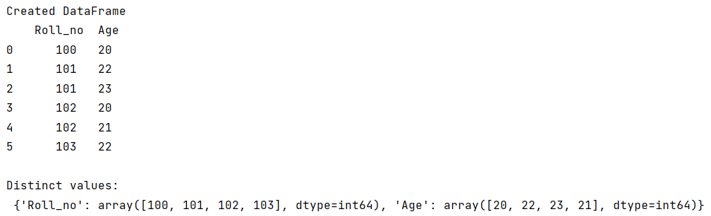# How to select distinct across multiple DataFrame columns in pandas?

Given a Pandas DataFrame, we have to select distinct across multiple columns. By Pranit Sharma Last updated : September 22, 2023

Distinct elements are those elements that are not similar to other elements, in other words, we can say that distinct elements are those elements that have their occurrence 1.

## Selecting distinct across multiple DataFrame columns

To select distinct elements across multiple DataFrame columns, we need to check if there are any duplicates in the DataFrame or not and if there is any duplicate then we need to drop that particular value to select the distinct value. For this purpose, we will use DataFrame['col'].unique() method, it will drop all the duplicates, and ultimately we will be having all the distinct values as a result.

Note

To work with pandas, we need to import pandas package first, below is the syntax:

```import pandas as pd
```

Let us understand with the help of an example,

## Python program to select distinct across multiple DataFrame columns in pandas

```# Importing pandas package
import pandas as pd

# Creating am empty dictionary
d = {}

# Creating a DataFrame
df = pd.DataFrame({
'Roll_no':[100,101,101,102,102,103],
'Age':[20,22,23,20,21,22]
})

# Display DataFrame
print("Created DataFrame\n",df,"\n")

# Drop duplicates
for col in df.columns:
d[col]=df[col].unique()

# Display result
print("Distinct values:\n",d)
```

### Output

The output of the above program is: